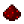# fs.combineFunction fs.combine Combines two path components, returning a path consisting of the local path nested inside the base path. The resultant path consists of all the components of localPath inside all the components of basePath. Neither path needs to exist; this function only manipulates strings and does not touch the filesystem. Syntax fs.combine(string basePath, string localPath) Returns string the combined path Part of ComputerCraft API fs

## ExamplesExample Computes the absolute path to a file called "d" inside a directory called "c", where "c" is itself inside a directory "b" which is inside a directory "a" Code ```print(fs.combine("a/b", "c/d")) ``` Output a/b/c/dExample An empty first path refers to the root directory, so the second path is returned Code ```print(fs.combine("", "c/d")) ``` Output c/dExample An empty second path refers to the first path directly, so the first path is returned Code ```print(fs.combine("a/b", "")) ``` Output a/bExample The last two examples make sense combined, returning the empty string (referring to the root directory) Code ```print(fs.combine("", "")) ```